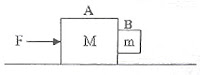## Wednesday, May 23, 2007

### A Question Involving Inertial Force and Frictional ForceHere is a question which requires your understanding of inertial force and frictional force:

Two bodies A and B of masses ‘M’ and ‘m’ respectively move together under the action of a horizontal force ‘F’ as shown in the figure. The body of mass A is sliding on a horizontal frictionless surface. If the coefficient of friction between A and B is μ, B will not move down under its own weight if F is at least

(a) Mg/μm (b) (M+m)g/μm (c) mg (d) mg/μ (e) (M+m)g/μ

There is frictional force μma between the bodies A and B since there is a normal force equal to the inertial force ma which the body B exerts on A.

The acceleration ‘a’ of the bodies under the action of the force F is given by a = F/(M+m)

Therefore, frictional force between A and B = μma = μmF/(M+m).

[Note that the maximum value of the frictional force is equal to mg since it is a self adjusting force just sufficient to balance the weight mg of the body B and to prevent the relative motion between A and B].

The least value of F is given by μmF/(M+m) = mg so that F = (M+m)g/μ

Now, suppose the force F is less than the least value required to prevent the body B from sliding down. If it slides down with acceleration ‘a1’ and the coefficient of kinetic friction is μk what is the value of F?

[In the question we discussed above, the coefficient of friction (μ) we used is the coefficient of static friction, which is the one having the maximum possible value. When the body slides down, the frictional force is lowered and the coefficient of sliding friction therefore has a smaller value. You may be remembering that the least coefficient is the coefficient of rolling friction]

The frictional force when B slides down is μkma so that the net downward force on B is

mg μkma. The downward acceleration therefore is (mgμkma)/m = (g μka)

Therefore we have g μka = a1

Since a = F/(M+m), we have g μkF/(M+m) = a1, from which F = (M+m)(g–a1)/μk